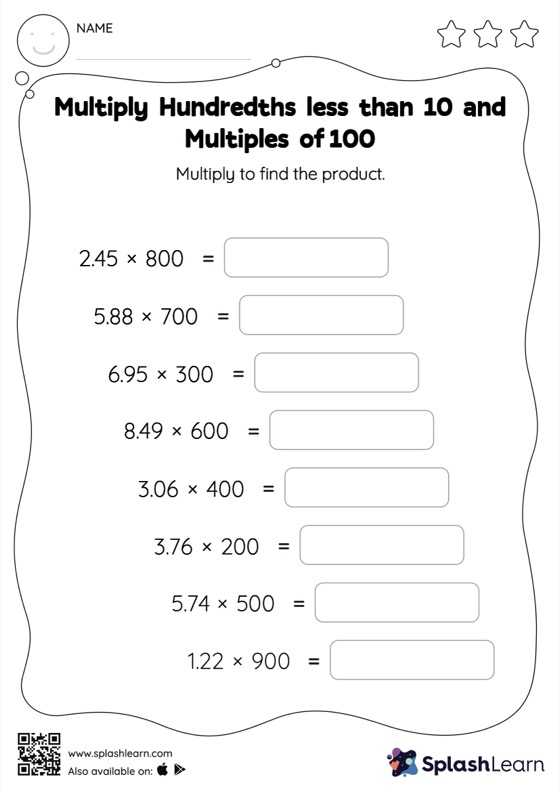# Multiply Hundredths less than 10 and Multiples of 100: Horizontal Multiplication Worksheet

Home > Multiply Hundredths less than 10 and Multiples of 100: Horizontal MultiplicationTask your little mathematicians to crack the code of multiplying hundredths less than 10 and multiples of 100 with this fun worksheet. This worksheet is about practicing with the horizontal format in which numbers are written side by side. To develop flexibility with numbers and operations, students need to have enough practice in this format and not just rely on the vertical/column method.